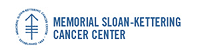Memorial Sloan-Kettering Cancer Center, Dept. of Epidemiology & Biostatistics Working Paper Series

Abstract

Rationale and Objectives: ROC curves are ubiquitous in the analysis of imaging metrics as markers of both diagnosis and prognosis. While empirical estimation of ROC curves remains the most popular method, there are several reasons to consider smooth estimates based on a parametric model.

Materials and Methods: A mixture model is considered for modeling the distribution of the marker in the diseased population motivated by the biological observation that there is more heterogeneity in the diseased population than there is in the normal one. It is shown that this model results in an analytically tractable ROC curve which is itself a mixture of ROC curves.

Results: The use of CK-BB isoenzyme in diagnosis of severe head trauma is used as an example. ROC curves are fit using the direct binormal method, ROCKIT and the Box-Cox transformation as well as the proposed mixture model. The mixture model generates an ROC curve that is much closer to the empirical one than the other methods considered.

Conclusions: Mixtures of ROC curves can be helpful in fitting smooth ROC curves in datasets where the diseased population has higher variability than can be explained by a single distribution.

Biostatistics

COinS